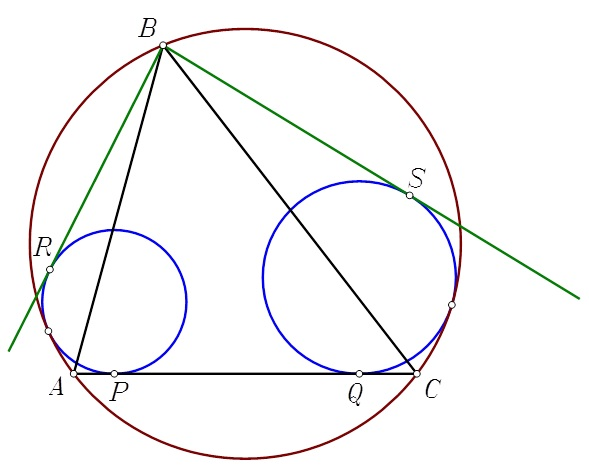# A lot of tangency points

Geometry Level 4

The triangle $ABC$ is inscribed in the circle $\mathcal{C}$. Circle $\mathcal{C}_1$ is tangent to the minor arc $AB$ of $\mathcal{C}$ and to side $AC$ at point $P$. Circle $\mathcal{C}_2$ is tangent to the minor arc $CB$ of $\mathcal{C}$ and to side $AC$ at point $Q$. From point $B$ draw the tangents $BR$ and $BS$ to circles $\mathcal{C}_1$ and $\mathcal{C}_2$, respectively. It is known that $BA=22$, $BC=23$, $BR=16$, $BS=17$, $PQ=15$. $AC$ can be written as $\frac{a}{b}$, where $a$ and $b$ are coprime positive integers. What is the value of $a+b$?×

Problem Loading...

Note Loading...

Set Loading...